Courses
Courses for Kids
Free study material
Free LIVE classes
More

# NEET Important Questions for Class 12 Chemistry AminesLIVE
Join Vedantu’s FREE Mastercalss

## Crack NEET with Ease By Solving Class 12 Chemistry Amines Important Questions

The chapter on Amines is quite an important topic introduced to students of Class 12 when they study Organic Chemistry. This chapter holds specific importance because of its inclusion in the syllabus of NEET. For students who want to score high marks in their NEET entrance examination, it is important to get their concepts clear about this chapter. This is where Amines Class 12 important questions and answers will be able to help them out. In order to gain an elaborate understanding of the chapter, it is crucial for students to attempt these important questions that have been formulated by the subject matter experts at Vedantu.

By solving these questions, students will truly be able to understand what the chapter entails. Not to mention that they will have enough practice to memorise the contents of the topic. With a thorough understanding of the chapter and the concepts in it, students will be more confident to appear for the NEET entrance exams. Download the important questions and solutions right now from Vedantu and include them in your study material to get the best benefits.

Last updated date: 22nd Sep 2023
Total views: 139.5k
Views today: 3.39k

## Multiple Choice Type

1. Amongst the following, the strongest base in aqueous medium is

(NCERT Exemplar)

$\begin{array}{*{20}{l}}{\left( {\rm{A}} \right){\rm{ C}}{{\rm{H}}_{\rm{3}}}{\rm{N}}{{\rm{H}}_{\rm{2}}}}\\{\left( {\rm{B}} \right){\rm{ NCC}}{{\rm{H}}_{\rm{2}}}{\rm{N}}{{\rm{H}}_{\rm{2}}}}\\{\left( {\rm{C}} \right){\rm{ }}{{\left( {{\rm{C}}{{\rm{H}}_{\rm{3}}}} \right)}_{\rm{2}}}{\rm{NH}}}\\{\left( {\rm{D}} \right){\rm{ }}{{\rm{C}}_{\rm{6}}}{{\rm{H}}_{\rm{5}}}{\rm{NHC}}{{\rm{H}}_{\rm{3}}}}\end{array}$

2. Which of the following is the weakest Bronsted base?

(NCERT Exemplar)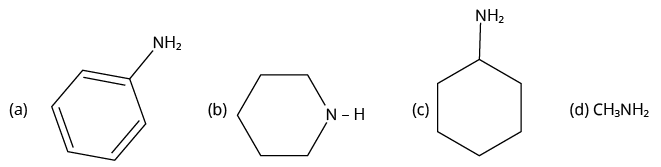Amines

3. Which one of the following gives the most stable Diazonium salt?

(JEE MAIN 2022)

(A) ${\rm{C}}{{\rm{H}}_{\rm{3}}}{\rm{ - C}}{{\rm{H}}_{\rm{2}}}{\rm{ - C}}{{\rm{H}}_{\rm{2}}}{\rm{ - N}}{{\rm{H}}_{\rm{2}}}$

(B)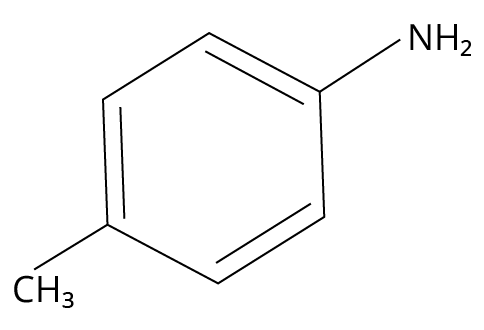4-methylbenzylamine

(C)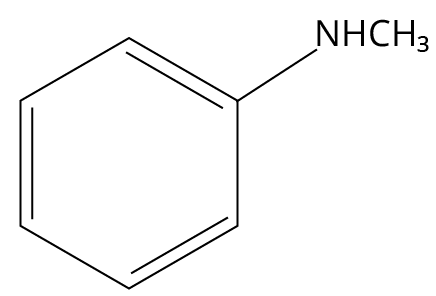N-methylbenzylamine

(D)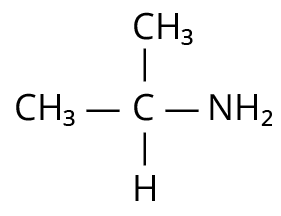Isopropylamine

4. Which of the following is not a correct statement for primary aliphatic amines?

(JEE MAIN 2021)

(A) The intermolecular association in primary amines is less than the intermolecular association in secondary amines.

(B) Primary amines are treated with nitrous acid solution from corresponding alcohols except methyl amines.

(C) Primary amines are less basic than the secondary amines.

(D) Primary amines can be prepared by the Gabriel phthalimide synthesis.

5. Given below are two statements:
Statement I: Aniline is less basic than acetamide.
Statement II: In aniline, the lone pair of electrons on a nitrogen atom is delocalized over a benzene ring due to resonance and hence less available to a proton.
Choose the most appropriate option;

(JEE MAIN 2021)

(A) Statement I is true but statement II is false.

(B) Statement I is false but statement II is true.

(C) Both statement I and statement II are true.

(D) Both statement I and statement II are false.

6. A reaction of benzonitrile with one equivalent CH3MgBr followed by hydrolysis produces a yellow liquid "P". The compound "P" will give positive _________.

(JEE MAIN 2021)

(A) Iodoform test

(B) Schiff’s test

(C) Ninhydrin’s test

(D) Tollen’s test

7. In Carius method, halogen containing organic compound is heated with fuming nitric acid in the presence of:

(JEE MAIN 2021)

(A) ${\rm{HN}}{{\rm{O}}_{\rm{3}}}$

(B) ${\rm{AgN}}{{\rm{O}}_{\rm{3}}}$

(C) ${\rm{CuS}}{{\rm{O}}_{\rm{4}}}$

(D) ${\rm{BaS}}{{\rm{O}}_{\rm{4}}}$

8. Compound A is converted to B on reaction with CHCl3 and KOH. The compound B is toxic and can be decomposed by C. A, B and C respectively are:

(JEE MAIN 2021)

(A) Primary amine, nitrile compound, conc. HCl

(B) Secondary amine, isonitrile compound, conc. NaOH

(C) Primary amine, isonitrile compound, conc. HCl

(D) Secondary amine, nitrile compound, conc. NaOH

9. In the reaction of hypobromite with amide, the carbonyl carbon is lost as:

(JEE MAIN 2021)

(A) ${\rm{C}}{{\rm{O}}_{\rm{2}}}$

(B) CO

(C) ${\rm{HC}}{{\rm{O}}_{\rm{3}}}^ -$

(D) ${\rm{C}}{{\rm{O}}_{\rm{3}}}^{2 - }$

10. Ammonolysis of Alkyl halides followed by the treatment with NaOH solution can be used to prepare primary, secondary and tertiary amines. The purpose of NaOH in the reaction is :

(JEE MAIN 2021)

(A) to remove acidic impurities

(B) to remove basic impurities

(C) to activate ${\rm{N}}{{\rm{H}}_{\rm{3}}}$used in the reaction

(D) to increase the reactivity of alkyl halide

11. Given below are two statements :
Statement I: A mixture of chloroform and aniline can be separated by simple distillation.
Statement II: When separating aniline from a mixture of aniline and water by steam distillation aniline boils below its boiling point.
In the light of the above statements, choose the most appropriate answer from the options given below :

(JEE MAIN 2021)

(A) Both statement I and statement II are true.

(B) Both statement I and statement II are false.

(C) Statement I is true but statement II is false.

(D) Statement I is false but statement II is true.

12. The most appropriate reagent for conversion of C2H5CN into CH3CH2CH2NH2 is

(JEE MAIN 2020)

(A) ${\rm{NaB}}{{\rm{H}}_{\rm{4}}}$

(B) ${\rm{Ca}}{{\rm{H}}_{\rm{2}}}$

(C) ${\rm{Na}}\left( {{\rm{CN}}} \right){\rm{B}}{{\rm{H}}_{\rm{3}}}$

(D) ${\rm{LiAl}}{{\rm{H}}_{\rm{4}}}$

13. Which of the following will react with ${\rm{CHC}}{{\rm{l}}_{\rm{3}}}{\rm{\; + alc}}{\rm{. KOH?}}$

(JEE MAIN 2020)

(B) Thymine and proline

14. Ethylamine $\left( {{{\rm{C}}_{\rm{2}}}{{\rm{H}}_{\rm{5}}}{\rm{N}}{{\rm{H}}_{\rm{2}}}} \right)$ can be obtained from N-ethylphatalimide on treatment with :

(JEE MAIN 2019)

(A) ${\rm{Ca}}{{\rm{H}}_{\rm{2}}}$

(B) ${{\rm{H}}_{\rm{2}}}{\rm{O}}$

(C) ${\rm{NaB}}{{\rm{H}}_{\rm{4}}}$

(D) ${\rm{N}}{{\rm{H}}_{\rm{2}}}{\rm{N}}{{\rm{H}}_{\rm{2}}}$

15. A compound 'X' on treatment with ${\rm{B}}{{\rm{r}}_{\rm{2}}}{\rm{/NaOH,}}$ provided ${{\rm{C}}_{\rm{3}}}{{\rm{H}}_{\rm{9}}}{\rm{N,}}$ which gives positive carbylamine test. Compound 'X' is :

(A) ${\rm{C}}{{\rm{H}}_{\rm{3}}}{\rm{C}}{{\rm{H}}_{\rm{2}}}{\rm{C}}{{\rm{H}}_{\rm{2}}}{\rm{CON}}{{\rm{H}}_{\rm{2}}}$

(B) ${\rm{C}}{{\rm{H}}_{\rm{3}}}{\rm{CON}}{\left( {{\rm{C}}{{\rm{H}}_{\rm{3}}}} \right)_{\rm{2}}}$

(C) ${\rm{C}}{{\rm{H}}_{\rm{3}}}{\rm{C}}{{\rm{H}}_{\rm{2}}}{\rm{COC}}{{\rm{H}}_{\rm{2}}}{\rm{NH}}$

(D) ${\rm{C}}{{\rm{H}}_{\rm{3}}}{\rm{COC}}{{\rm{H}}_{\rm{2}}}{\rm{NHC}}{{\rm{H}}_{\rm{3}}}$

## Multiple Choice Type

16. Which of the following cannot be prepared by Sandmeyer’s reaction?

(NCERT Exemplar)

(A) Chlorobenzene

(B) Bromobenzene

(C) Iodobenzene

(D) Fluorobenzene

17. The reagents that can be used to convert benzene diazonium chloride to

benzene are

(NCERT Exemplar)

$\begin{array}{*{20}{l}}{\left( {\rm{A}} \right){\rm{ SnC}}{{\rm{l}}_{\rm{2}}}{\rm{/HCl}}}\\{\left( {\rm{B}} \right){\rm{ C}}{{\rm{H}}_{\rm{3}}}{\rm{C}}{{\rm{H}}_{\rm{2}}}{\rm{OH}}}\\{\left( {\rm{C}} \right){\rm{ }}{{\rm{H}}_{\rm{3}}}{\rm{P}}{{\rm{O}}_{\rm{2}}}}\\{\left( {\rm{D}} \right){\rm{ LiAl}}{{\rm{H}}_{\rm{4}}}}\end{array}$

18. Under which of the following reaction conditions, aniline gives p-nitro derivative as the major product?

(NCERT Exemplar)

(A) Acetyl chloride/pyridine followed by reaction with ${\rm{conc}}{\rm{. }}{{\rm{H}}_{\rm{2}}}{\rm{S}}{{\rm{O}}_{\rm{4}}}{\rm{ + conc}}{\rm{. HN}}{{\rm{O}}_{\rm{3}}}$

(B) Acetic anhydride/pyridine followed by ${\rm{conc}}{\rm{. }}{{\rm{H}}_{\rm{2}}}{\rm{S}}{{\rm{O}}_{\rm{4}}}{\rm{ + conc}}{\rm{. HN}}{{\rm{O}}_{\rm{3}}}$

(C) Dil. ${\rm{HCl}}$followed by reaction with ${\rm{conc}}{\rm{. }}{{\rm{H}}_{\rm{2}}}{\rm{S}}{{\rm{O}}_{\rm{4}}}{\rm{ + conc}}{\rm{. HN}}{{\rm{O}}_{\rm{3}}}$

(D) Reaction with ${\rm{conc}}{\rm{. }}{{\rm{H}}_{\rm{2}}}{\rm{S}}{{\rm{O}}_{\rm{4}}}{\rm{ + conc}}{\rm{. HN}}{{\rm{O}}_{\rm{3}}}$

19. Which of the following reactions are correct?

(NCERT Exemplar)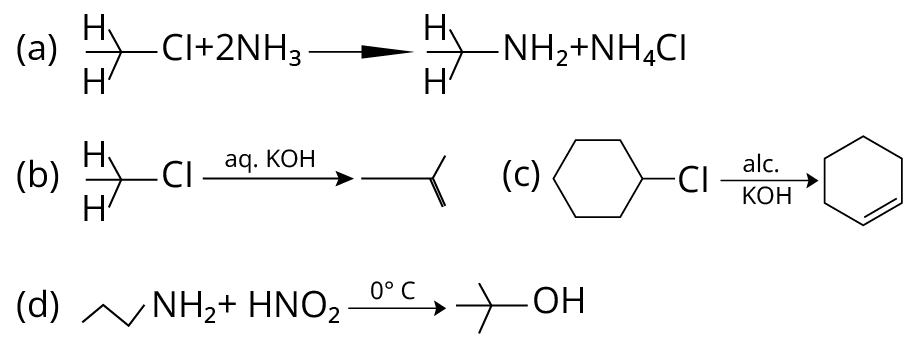Reactions of Amino Compounds

## Numerical Type

20. The total number of reagents from those given below, that can convert nitrobenzene into aniline is ___________. (Integer answer)
I. Sn  HCl
II. Sn  NH4OH
III. Fe  HCl
IV. Zn  HCl
V. H2  Pd
VI. H2  Raney Nickel

(JEE Main 2021)

21. The total number of amines among the following which can be synthesised by Gabriel synthesis is __________.

(JEE MAIN 2021)

22. The mass percentage of nitrogen in histamine is _____.

(JEE MAIN 2020)

23. The number of chiral centres present in [B] is _____.

(JEE MAIN 2020)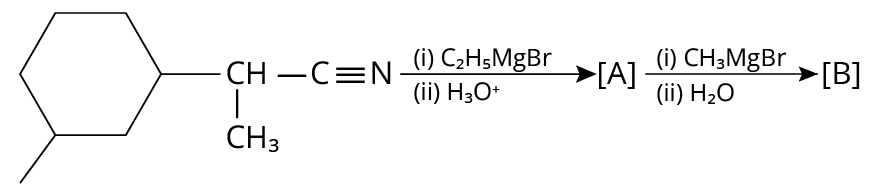Reactions of Aromatic Amino Compounds

24. In gaseous triethylamine the "CNC" bond angle is _________ degree.

(JEE MAIN 2021)

25. The number of nitrogen atoms in a semicarbazone molecule of acetone is _____________

(JEE MAIN 2021)

## Solutions

1. (C)

The general trend of Basicity of amines is in order- ${{\rm{3}}^{\rm{o}}}{\rm{ > }}{{\rm{2}}^{\rm{o}}}{\rm{ > }}{{\rm{1}}^{\rm{o}}}$

As (C) is an secondary amine, so it will be more basic than ${\rm{C}}{{\rm{H}}_{\rm{3}}}{\rm{N}}{{\rm{H}}_{\rm{2}}}$ which is the primary amine. In ${{\rm{C}}_{\rm{6}}}{{\rm{H}}_{\rm{5}}}{\rm{CHN}}{{\rm{H}}_{\rm{3}}}$, delocalisation of lone pairs of electrons occurs on the n atom into the benzene ring. Also, due to the -I effect of the ${\rm{CN}}$ group in (B), it is less basic than (C)

Overall from the given options, (C) is the list basic amine.

2. (A)

When an amine group ${\rm{N}}{{\rm{H}}_{\rm{2}}}$ is attached to a benzene ring, lone pairs of nitrogen electrons delocalize into the benzene ring and are no longer available for donation. As a result, they are the weakest base.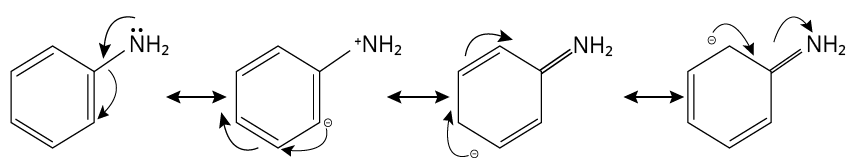Aniline

Thus, option (A) is correct.

3. (B)

Primary aromatic amines would be able to give the most stable diazonium salt in the presence of nitrous acid.

The reaction is shown below.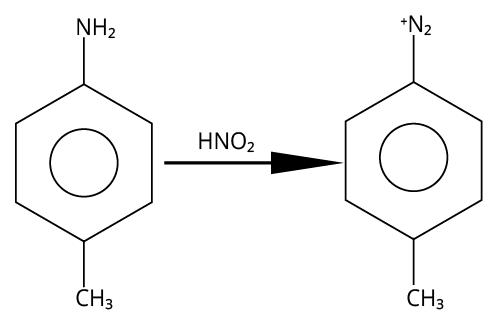Reaction of 4-methylaniline

4. (A)

Due to the availability of two hydrogen atoms, the intermolecular interaction is more pronounced in primary amines compared to secondary amines.

5. (B)

Since the lone pair of nitrogen in acetamide is delocalized to the more electronegative element oxygen, aniline is more basic than acetamide.

The nitrogen lone pair delocalized over the benzene ring in aniline.

6. (A)

A reaction of benzonitrile with one equivalent CH3MgBr followed by hydrolysis is shown below.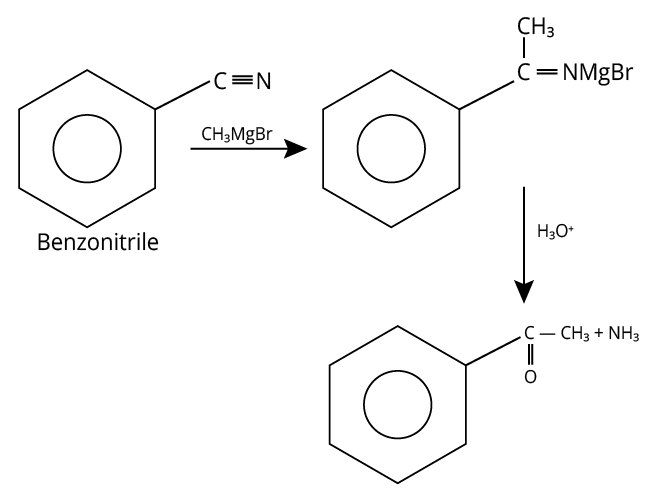Hydrolysis of Benzonitrile

The compound formed other than amine in the product is a yellow liquid “P” and it gives the positive iodoform test.

7. (B)

The carius method involves heating an organic molecule with fuming nitric acid while silver nitrate is present.

As a reagent, lunar caustic $\left( {{\rm{AgN}}{{\rm{O}}_{\rm{3}}}} \right)$ is used to identify Cl, Br, and I ions respectively.

8. (C)

When primary amine combines with chloroform, poisonous isonitrile is produced. When isonitrile then reacts with ${\rm{HCl}}/{{\rm{H}}_{\rm{3}}}{{\rm{O}}^{\rm{ + }}}{\rm{,}}$ primary amine and acid are produced.

Hence, A-primary amine, B-isonitrile compound and C-conc. HCl.

9. (D)

The reaction of hypobromite with amide is shown below.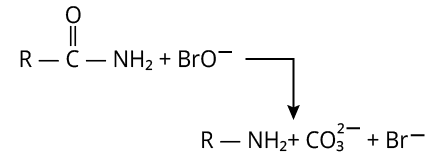Reaction of Amide with Hypobromite

Here, carbonyl carbon is lost as ${\rm{C}}{{\rm{O}}_{\rm{3}}}^{2 - }$.

10. (A)

The complete reaction is shown below.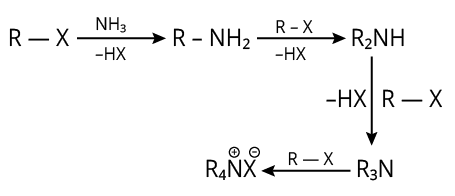Reaction of Alkyl Halide with Amine

The reaction produces HX (acid).

So, to get rid of these acidic contaminants, we utilise NaOH.

11. (A)

Simple distillation can be used to separate a mixture of aniline and chloroform because their boiling points are sufficiently different.

Aniline (b.p. 334 K) , and chloroform (b.p. 457 K)

In steam distillation, the mixture will boil close to but below 373 K if one of the ingredients is water and the other is a water-insoluble chemical (like aniline).

12. (D)

13. (C)

Primary online from adenine and lysine both react with ${\rm{CHC}}{{\rm{l}}_{\rm{3}}}{\rm{\; + alc}}{\rm{. KOH}}{\rm{.}}$

The formula of adenine and lysine are shown below.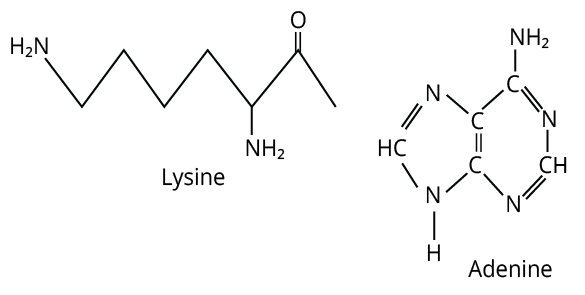14. (D)

After being treated with NH2NH2, N-ethyl phthalimide produces ethylamine. The Galbriel phatalimide manufacturing reaction ends with this stage.

15. (A)

A primary amine with one fewer carbon atom than the original amide is created by the ${\rm{B}}{{\rm{r}}_{\rm{2}}}/{\rm{NaOH}}$ (Hoffman Hypobromide reagent), which produces the carbylamine test. There should be ${\rm{ - CON}}{{\rm{H}}_{\rm{2}}}$ group in the amine that produces primary amine.

16. (C), (D)

Sandmeyer's reaction makes it simple to prepare chloro and bromo arenes. Simply warming the diazonium salt solution with aqueous KI solution yields iodoarenes.

The Balz-Schiemann reaction is used to synthesise fluoroarenes.

All of the other reagents produce aniline. Thus, option (c) and (d) are correct.

17. (B), (C)

Mild reducing agents given in option B and C convert the benzene diazonium chloride into benzene while the reagents given in options a and d are strong reducing agents, they produce ${{\rm{C}}_{\rm{6}}}{{\rm{H}}_{\rm{5}}}{\rm{NHN}}{{\rm{H}}_{\rm{2}}}$.

The reactions are shown below.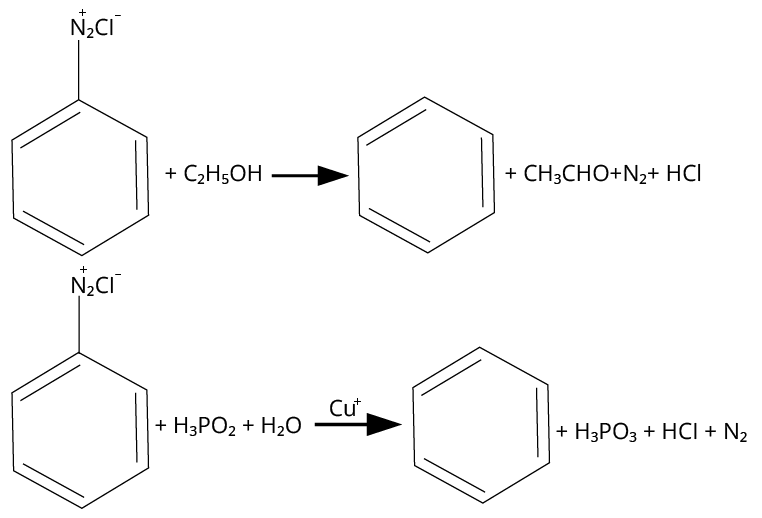Reactions of Diazonium Salt with Different Reagents

Thus, option (B) and (C) are correct.

18. (A), (B)

Aniline is acetylated when it reacts with acetyl chloride or acetic anhydride in the presence of pyridine base. On treatment with concentrated sulfuric acid and nitric acid, the N-acetyl aniline formed is ortho para directing group, which guides the further reagent to attack on the para position and produce p-nitroaniline.

The reaction is shown below.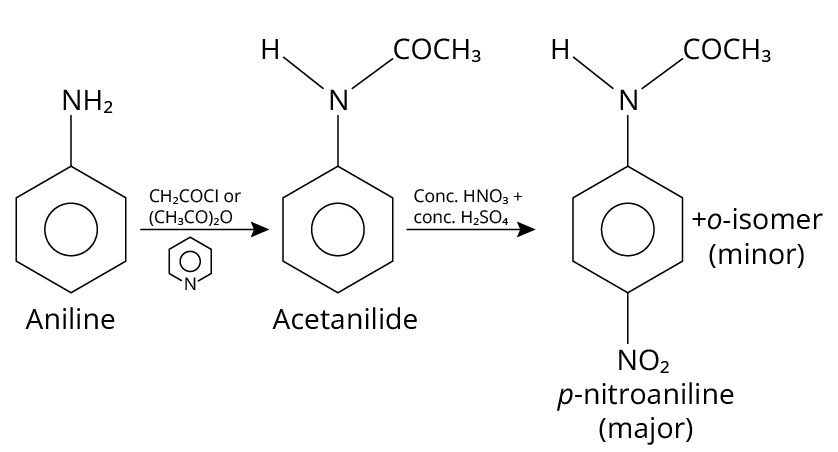Reaction of Aniline

Thus, option (A) and (B) are correct.

19. (A), (C)

(A) Primary alkyl halides react with ammonia to give primary amines. So, option (A) is correct.

(B) Elimination process doesn’t occur with ${\rm{aq}}{\rm{. KOH}}$, with ${\rm{aq}}{\rm{. KOH}}$, hydrolysis process takes place. So, (B) is incorrect

(C) Dehydrohalogenation i.e. elimination of ${\rm{HCl}}$ occurs which produces alkene as product takes place with ${\rm{alc}}{\rm{.KOH}}$ (C) is correct

(D) In this reaction, aliphatic primary amines, on treatment with nitrous acid, produce primary alcohol as a product.

Therefore (D) is incorrect.

20. 5

The reagents that can convert nitrobenzene to aniline are shown below.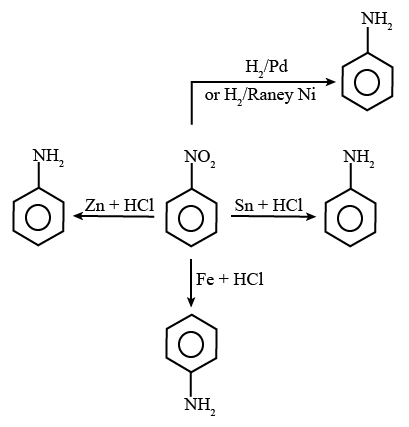Reaction of Aniline with Different Reagents

(i) Sn + HCl
(ii) Fe + HCl
(iii) Zn + HCl
(iv) H2  Pd
(v) H2 (Raney Ni)

The total number of reagents from those given below, that can convert nitrobenzene into aniline is 5

21. 3

One aliphatic or alicyclic amine is made using the Gabriel phthalimide synthesis. As a result, the amine that can be produced using the Gabriel phthalimide synthesis technique contains alpha -carbon.

Since aniline lacks alpha-C, the Gabriel reaction cannot be used to create it.

The remaining amines all have alpha-C in the appropriate location, making them capable of producing the Gabriel phthalimide reaction with ease.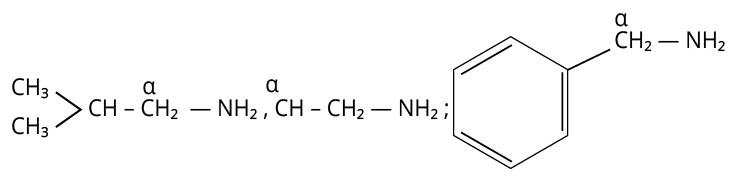Amines

22. 37.80

The structure of histamine is shown below.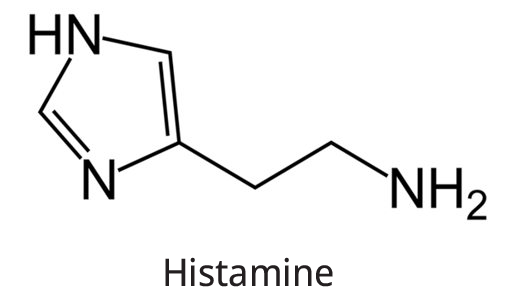Histidine

The chemical formula of Histamine is ${{\rm{C}}_{\rm{5}}}{{\rm{H}}_{\rm{9}}}{{\rm{N}}_{\rm{3}}}.$

So, the percentage by mass of N can be calculated by the formula,

$\% {\rm{ by mass}} = \frac{{3 \times {\rm{Atomic~mass~ of~ N}}}}{{{\rm{9}} \times {\rm{Atomic~mass~of~H}} + {\rm{5}} \times {\rm{Atomic~mass~of~C}} + {\rm{3}} \times {\rm{Atomic~mass~of~N}}}} \times 100$

Substitute all the known values,

$\begin{array}{c}\% {\rm{ by mass}} = \frac{{3 \times {\rm{14}}}}{{{\rm{9}} \times {\rm{1}} + {\rm{5}} \times {\rm{12}} + {\rm{3}} \times 14}} \times 100\\ = \frac{{42}}{{111}} \times 100\\ = 37.84\% \end{array}$

23. 4

The complete reaction is shown below.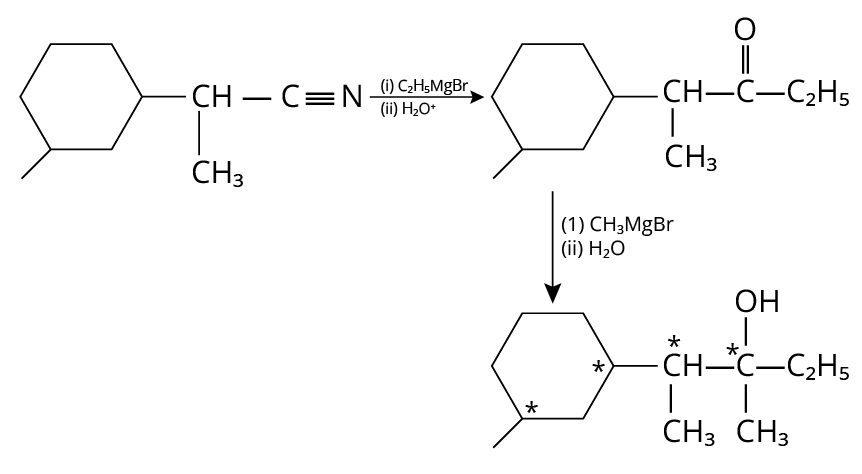Reduction of Amine

In the final products, there are four chiral carbons present.

24. 180

In triethylamine, the central atoms (nitrogen) contain 3 bond pairs and 1 lone pair. So, according to the VSEPR theory, the hybridization of nitrogen is $s{p^3}$ and bond angle is ${180^ \circ }.$

Pyramidal shape of triethylamine: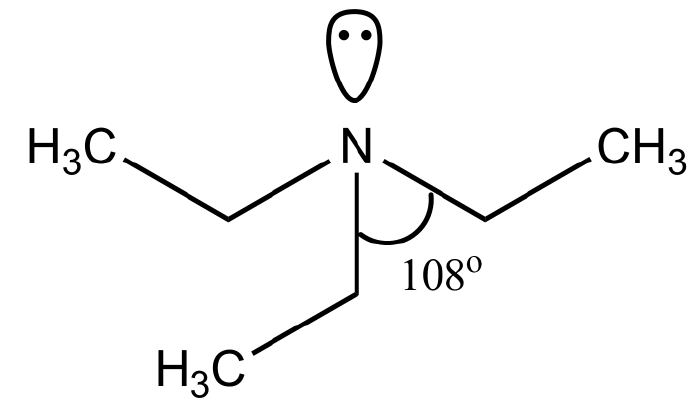Triethylamine

25. 3

The structure of semicarbazone is shown below.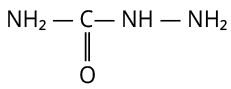Semicarbazone

Here. three nitrogen atoms are present.

Importance of Chemistry Chapter Amines for NEET Preparation

Students who are preparing for the NEET entrance examination will have to study the chapter of Amines which is the 14th chapter in the Class 12 syllabus. By studying the chapter properly, they will be able to answer the questions related to the chapter. NEET students need to know about the important topics in Amines for NEET in order to prepare the chapter well and score high marks in the examination.

The chapter introduces the students to the term Amines which are organic compounds containing ammonia that have one or more aryl or alkyl groups that are attached to an atom of nitrogen. As they progress through the chapter, students will be able to learn about the different characteristics of Amines and how they are represented.

Students will also learn how amines can be classified into Primary, Secondary, and Tertiary Amines on the basis of the Nitrogen atoms that are attached to the groups. They will learn the structure representation of amines and along with that, they will see all the natural examples of amines that tend to exist.

Further in the chapter, students will learn the physical and chemical properties of amines such as Boiling Point, State, Dipole Moment, and Solubility. They will be acquainted with different methods used for the preparation of amines such as Hoffmann’s Ammonolysis Method, Sabatier Reaction, Schmidt Reaction, Curtius Reaction, and so much more. These details will help them gain a better understanding of the chapter. By preparing the important questions and answers for the Amines chapter, students will be able to tackle questions from the NEET examination easily.

## Benefits of Vedantu’s Chemistry Amines Important Questions

The important questions have been prepared by the subject experts at Vedantu and hence they have all the accurate details and explanations in the solutions. Apart from that, the experts have used very simple language so that students have no trouble understanding the solutions.

Preparing for NEET becomes really easy as students will be able to practice more with the questions that have been provided to them. Solving more questions from the Amines Class 12 important questions PDF will enable them to understand the chapter efficiently and take the NEET exam confidently.

By thoroughly studying the question papers, students will be able to gather information about the examination pattern of NEET. They can figure out which topics are important from the chapter and focus more on those to perform well.

## Easy NEET Preparation with Important Questions for Amines

This is your chance to prepare well for the NEET examination by downloading Amines important questions PDF from Vedantu. Anyone visiting the official website will be able to get their hands on this incredible study material that is going to be the perfect aid in cracking the NEET entrance examination. Learn more about amines and solve tricky questions from the PDF files to enhance your knowledge about the chapter.

## FAQs on NEET Important Questions for Class 12 Chemistry Amines

1. Why amines are soluble in dilute HCL?

Receiving an H+ ion from the HCL, the amines become charged and hence form very strong ion-dipole interactions with the molecules of water.

2. Why doesn’t aniline dissolve in water?

The presence of a –C6H5 group which is hydrophobic in nature doesn’t allow aniline to form many hydrogen bonds with water. Hence, it remains insoluble.

3. Do amines have a lower boiling point compared to alcohols?

Yes, since nitrogen is less electronegative than oxygen, amines have a pretty low boiling point.

4. Why is alkylamine more basic than ammonia?

An alkylamine is more basic than ammonia due to the electron releasing inductive effectiveness of the alkyl group which causes an increase in the electron density of the nitrogen atom. Thus, it is able to donate an electron pair more easily than ammonia.